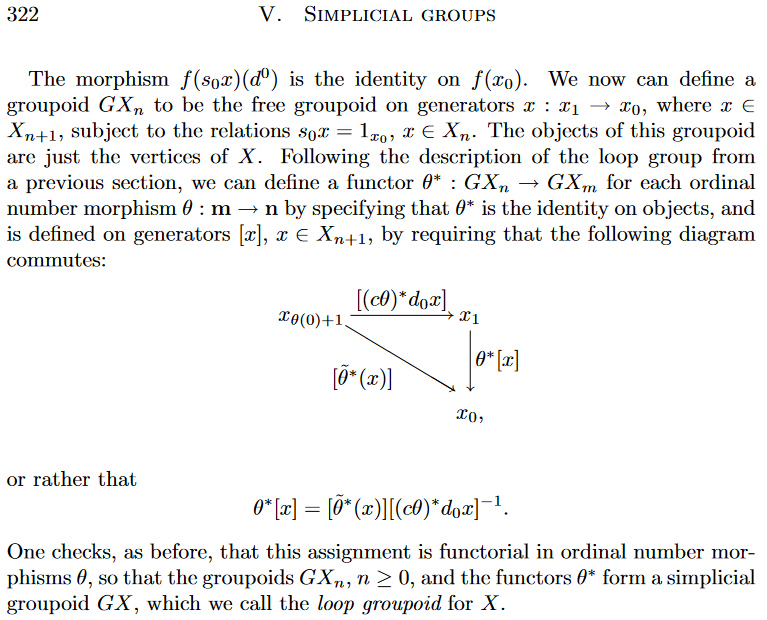# nLab Dwyer-Kan fundamental simplicial groupoid

Contents

### Context

#### Model category theory

Definitions

Morphisms

Universal constructions

Refinements

Producing new model structures

Presentation of $(\infty,1)$-categories

Model structures

for $\infty$-groupoids

for ∞-groupoids

for equivariant $\infty$-groupoids

for rational $\infty$-groupoids

for rational equivariant $\infty$-groupoids

for $n$-groupoids

for $\infty$-groups

for $\infty$-algebras

general $\infty$-algebras

specific $\infty$-algebras

for stable/spectrum objects

for $(\infty,1)$-categories

for stable $(\infty,1)$-categories

for $(\infty,1)$-operads

for $(n,r)$-categories

for $(\infty,1)$-sheaves / $\infty$-stacks

#### Enriched category theory

enriched category theory

# Contents

## Idea

The Dwyer-Kan fundamental simplicial groupoid (often “loop groupoid”) [Dwyer & Kan (1984), §3.1] of a simplicial set $K$ is the simplicial groupoid whose objects are the vertices of $K$ and whose hom-simplicial set between a pair of such picks up the composable sequences of higher dimensional simplices, where the zeroth vertex is thought of as the domain object and the first vertex as the codomain.

So the “loop”-terminology is a misnomer: It really refers to a simplicial version of a path groupoid-construction which may be thought of as modelling a fundamental $\infty$-groupoid as a simplicial groupoid.

But applied to the special case of a reduced simplicial set this construction reduces to the Kan loop group construction, which is properly a model for a loop space-construction.

The Dwyer-Kan groupoid construction is a left Quillen equivalence (right adjoint to a groupoidal simplicial classifying space-construction) from the classical model structure on simplicial sets to the model structure on simplicial groupoids [Dwyer & Kan (1984), Thm. 3.3], and as such exhibits the homotopy theory of simplicial groupoids as an equivalent incarnation of classical homotopy theory.

## Definitions

There are different choices of how to define a DK-type loop groupoid functor, depending, for instance, on which pair of vertices in the $n$-simplex of a simpilcial set are to be regarded as the source- and target-object of the corresponding morphism in the loop groupoid. In the original definition Dwyer & Kan (1984), §3.1 took the direction of the morphism to point from the $1$st to the 0th vertex, $1 \to 0$. But the modern convention in analogous constructions for Segal spaces etc. is to instead take $0 \to 1$.

Moreover, apparently the original definition in Dwyer & Kan (1984), §3.1 had a mistake in its definition of $d_0$ (according to Ehlers (1991), p. 10). Both issues are said to be fixed in Ehlers (1991), pp. 10.

Other definitions have been given, too (all necessarily isomorphic to each after their left adjointness to the simplicial classifying space-construction has been established, by uniqueness of adjoint).

In any case, the DK loop groupoid construction is a functor

$\mathcal{G} \colon sSet \longrightarrow sGrpd \,,$

which takes a simplicial set $S$ to a Dwyer-Kan simplicial groupoid (i.e. sSet-enriched groupoid) $\mathcal{G}(S)$.

### Original definition, fixed

The following is a slight modification of the original Dwyer & Kan (1984), §3.1, given by Ehlers (1991), pp. 10:

For $S \in sSet$ the simplicial groupoid $\mathcal{G}(S)$ is defined as follows:

• objects are the vertices of $S$:

$Obj\big(\mathcal{G}(K)\big) \,\coloneqq\, K_0$;

• morphisms in degree $n \in \mathbb{N}$ form the groupoid which is the quotient

$\array{ S_{n+1} &\overset{\phantom{----}}{\rightrightarrows} & S_0 \\ \mathllap{{}^{q}}\Big\downarrow && \Big\downarrow\mathrlap{{}^=} \\ \mathcal{G}(S)_n &\overset{\phantom{----}}{\rightrightarrows}& Obj\big(\mathcal{G}(S)\big) }$

of the free groupoid on the graph

$s,t \,\colon\, S_{n+1} \overset{\phantom{--}}{\rightrightarrows} S_0, \,,$
• with domain-function $s \coloneqq d_1 \circ d_2 \cdot d_3 \cdots d_{n+1}$,

and codomain-function $t \coloneqq d_0 \circ d_2 \cdot d_3 \cdots d_{n+1}$

by the relations

$q(s_0 \sigma_n) \,\sim\, id_{d_1 \cdots d_n \sigma_n}$, for all $\sigma_n \in S_n$.

• The face and degeneracy maps are given on generators by

• $d_0\big(q(\sigma)\big) \,\coloneqq\, q\big(d_1(\sigma_n)\big) \cdot q\big( d_0(\sigma_n)\big)^{-1}$

• $d_i\big(q(\sigma)\big) \,\coloneqq\,q\big(d_{i+1}(\sigma)\big)$ for $i \gt 0$

• $s_i\big(q(\sigma)\big) \,\coloneqq\,q\big(s_{i+1}(\sigma)\big)$ for $i \geq 0$.

Here is the definition given in Goerss & Jardine (1999) p. 322, (2009) p. 302:## Properties

### General

###### Remark

The fundamental simplicial groupoid functor has a right adjoint, $\overline{W}$ [Dwyer & Kan (1984) §3.2] also called the simplicial classifying space functor.

### Relation to rigidification of quasi-categories

Let

(1)$L \,\colon\, sSet\text{-}Cat \longrightarrow sSet\text{-}Grp$

denote the functor from sSet-enriched categories to sSet-enriched groupoids (Dwyer-Kan simplicial groupoids) which is degreewise given by the localization operation left adjoint to the full subcategory inclusion Grpd $\hookrightarrow$ Cat.

Let

(2)$\mathfrak{C} \,\colon\, sSet \longrightarrow sSet\text{-}Cat$

denote the operation of rigidification of quasi-categories.

###### Proposition

For $S \,\in\, sSet$ there is a natural transformation

$L \circ \mathfrak{C}(S) \longrightarrow \mathcal{G}(S)$

which is a Dwyer-Kan equivalence (from the localization (1) of the rigidification (2) to the Dwyer-Kan fundamental groupoid (as above).

[Minichiello, Rivera & Zeinalian (2023), Thm. 1.1 (Cor. 4.2)]

The original reference for the functor $G$ is Section 9 in

Later, Dwyer and Kan constructed the right adjoint $\bar W$ to $G$ in

Beware that the definition in Dwyer & Kan (1984) §3.1 apparently contains a mistake (in its formula for $d_0$). It may be that this problem was early on known and communicated by Joyal and Tierney, but in print the correction is given in:

• Philip Ehlers, pp. 10 in: Simplicial groupoids as models for homotopy type, Master’s thesis (1991) [pdf]